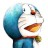# 杰拉斯的博客

## [ACM学习心得]关于sync_with_stdio(false);杰拉斯 | 时间：2012-03-27, Tue | 23,157 views

```#include<iostream>
using namespace std;
bool a;
int main()
{
int n, m, num, count;
while(scanf("%d%d",&n,&m)!=EOF){
memset(a, 0, sizeof(a));
for(int i=0; i<n; i++){
scanf("%d",&num);
a[num + 500000] = 1;
}
count = 0;
for(int j = 1000000; j >= 0; --j){
if(a[j]){
if(count == m - 1){
printf("%d\n",j-500000);
break;
}
printf("%d ",j-500000);
count++;
}
}
}
return 0;
}
```

```#include<iostream>
using namespace std;
bool a;
int main()
{
int n, m, num, count;
while(cin >> n >> m){
memset(a, 0, sizeof(a));
for(int i=0; i<n; i++){
cin >> num;
a[num + 500000] = 1;
}
count = 0;
for(int j = 1000000; j >= 0; --j){
if(a[j]){
if(count == m - 1){
cout << j - 500000 << endl;
break;
}
cout << j - 500000 << " ";
count++;
}
}
}
return 0;
}
```

`std::ios::sync_with_stdio(false);`